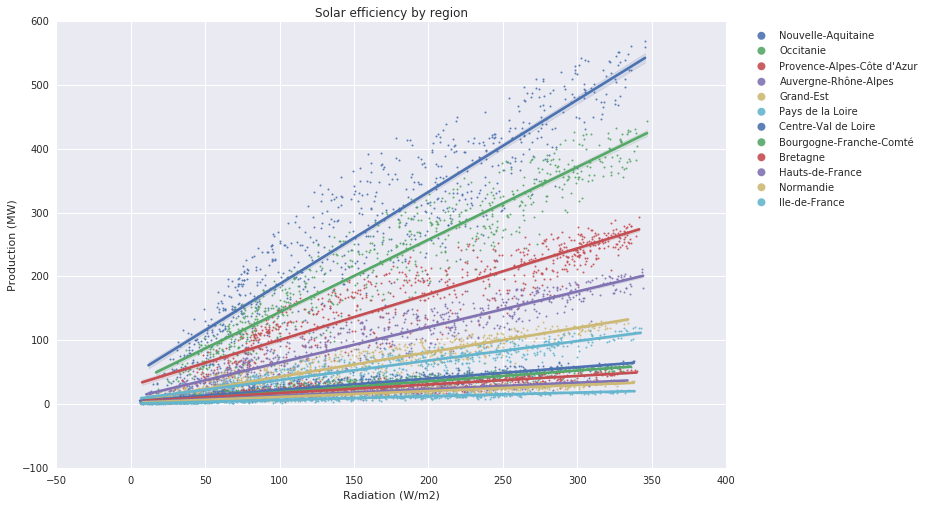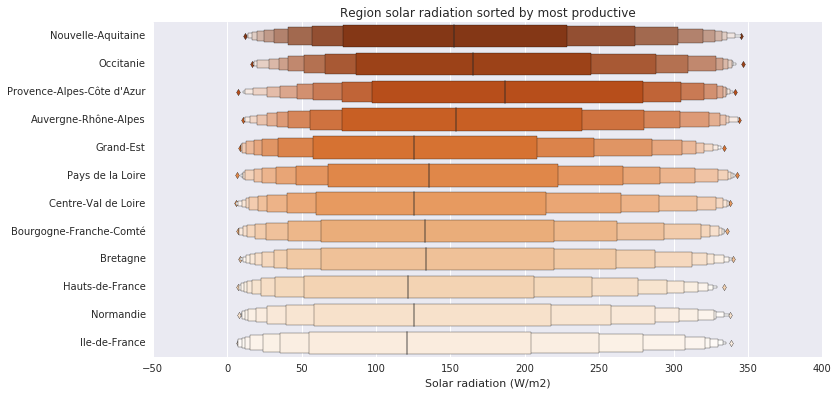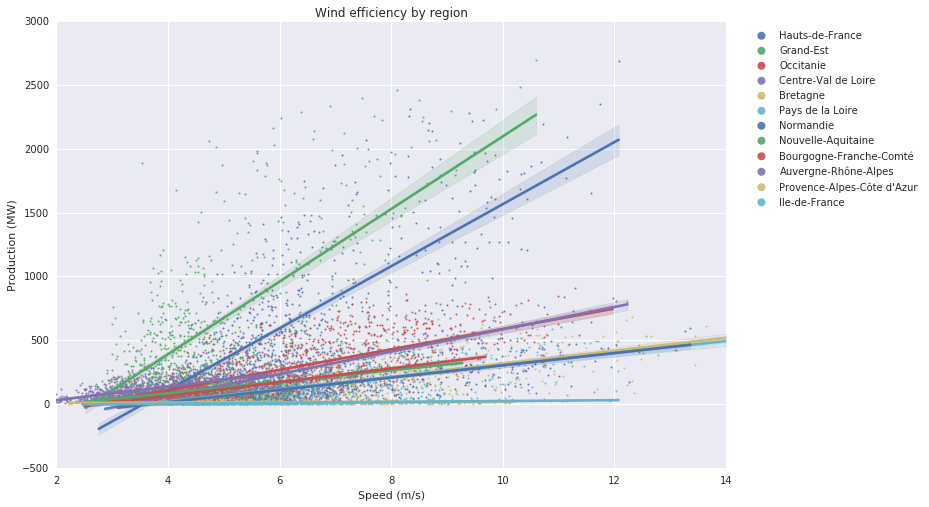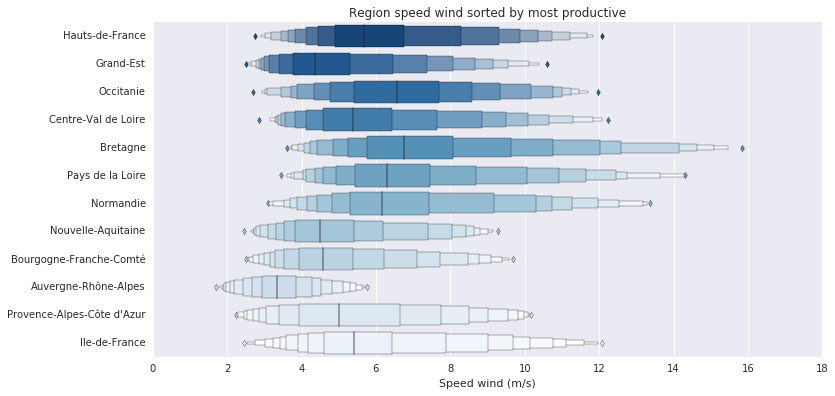# Analyze French Green Energies Production¶

Analyze french electricity green production. More analysis about french energy:

In [ ]:
import numpy as np
import pandas as pd
import datetime as dt
import matplotlib.pyplot as plt
import seaborn as sns

In :
%matplotlib inline
sns.set(style='darkgrid')


# Fetch data¶

In :
sw = pd.read_csv('rayonnement-solaire-vitesse-vent-tri-horaires-regionaux.csv',
sep=';',
parse_dates=)
sw.drop(['Code INSEE région'], axis=1, inplace=True)
sw.columns = ['date', 'region', 'wind_speed', 'solar_radiation']
sw.set_index('date', inplace=True)

In :
ecomix = pd.read_csv('eco2mix-regional-cons-def.csv', sep=';', parse_dates=)
ecomix.drop(['Code INSEE région', 'Nature', 'Date', 'Heure', 'Consommation (MW)', \
'Thermique (MW)', 'Nucléaire (MW)', 'Hydraulique (MW)', 'Pompage (MW)', \
'Bioénergies (MW)', 'Ech. physiques (MW)'], axis=1, inplace=True)
ecomix.columns = ['region', 'date', 'wind_prod', 'solar_prod']
ecomix.set_index('date', inplace=True)


# Analyze¶

## Solar performance analyze¶

In :
solar_prod = ecomix.drop('wind_prod', axis=1)
solar_prod = solar_prod.pivot_table(index=solar_prod.index,
values='solar_prod', columns='region')
solar_prod = solar_prod.groupby(by=pd.TimeGrouper('D')).mean()
solar_prod.columns = ['Auvergne-Rhône-Alpes', 'Bourgogne-Franche-Comté', 'Bretagne', \
'Centre-Val de Loire', 'Grand-Est', 'Hauts-de-France', 'Ile-de-France', \
'Normandie', 'Nouvelle-Aquitaine', 'Occitanie', 'Pays de la Loire', \
"Provence-Alpes-Côte d'Azur"]

start = dt.datetime(2016, 1, 1)
end = dt.datetime(2017, 12, 31)
solar_prod = solar_prod[start:end]

In :
solar = sw.drop('wind_speed', axis=1)
solar = solar.pivot_table(index=solar.index,
solar = solar.groupby(by=pd.TimeGrouper('D')).mean()
solar.drop('Corse', axis=1, inplace=True)

In :
solar_region_prod = solar_prod.mean().sort_values(ascending=False)

In :
fig, ax = plt.subplots(nrows=1, ncols=1, figsize=(12, 8))
for column in solar_region_prod.index:
sns.regplot(x=solar[column], y=solar_prod[column],
label=column, scatter_kws={"s": 4, "alpha": 0.9}, truncate=True, ax=ax)

ax.set_title('Solar efficiency by region')
ax.set_ylabel('Production (MW)')
ax.legend(bbox_to_anchor=(1.3, 1), markerscale=4)
plt.show()Solar production is very predicable. All clusters point are grouping arround a line for each region. According to the performance ratio by region (i.e. number of solar panel installed in the region), we can predict the production energy by the solar radiation.

In :
fig, ax = plt.subplots(ncols=1, nrows=1, figsize=(12, 6))
sns.lvplot(data=solar, orient='h', order=solar_region_prod.index,
palette='Oranges_r', scale='linear', ax=ax)

ax.set_title('Region solar radiation sorted by most productive')
ax.set_ylabel('')
plt.show()Do investments are well balanced over region ?
Yes, regions with the higher solar radiation are the most productive.

Howerver Ile-de-France's radiation is just 19% less than Nouvelle-Aquitaine's radiation but production is 3000% less. Investments are not as well balanced as expected, because they push up south regions and pull down north regions, whereas radiations oscillate only 33% in France.

## Wind performance analyze¶

In :
wind_prod = ecomix.drop('solar_prod', axis=1)
wind_prod = wind_prod.pivot_table(index=wind_prod.index, values='wind_prod', columns='region')
wind_prod = wind_prod.groupby(by=pd.TimeGrouper('D')).mean()
wind_prod.columns = ['Auvergne-Rhône-Alpes', 'Bourgogne-Franche-Comté', \
'Bretagne', 'Centre-Val de Loire', 'Grand-Est', \
'Hauts-de-France', 'Ile-de-France', 'Normandie', \
'Nouvelle-Aquitaine', 'Occitanie', 'Pays de la Loire', \
"Provence-Alpes-Côte d'Azur"]

start = dt.datetime(2016, 1, 1)
end = dt.datetime(2017, 12, 31)
wind_prod = wind_prod[start:end]

In :
wind = sw.drop('solar_radiation', axis=1)
wind = wind.pivot_table(index=wind.index, values='wind_speed', columns='region')
wind = wind.groupby(by=pd.TimeGrouper('D')).mean()
wind.drop('Corse', axis=1, inplace=True)

In :
wind_region_prod = wind_prod.mean().sort_values(ascending=False)

In :
fig, ax = plt.subplots(nrows=1, ncols=1, figsize=(12, 8))
for column in wind_region_prod.index:
sns.regplot(x=wind[column], y=wind_prod[column], label=column,
scatter_kws={"s": 4, "alpha": 0.9}, truncate=True, ax=ax)

ax.set_title('Wind efficiency by region')
ax.set_xlabel('Speed (m/s)')
ax.set_ylabel('Production (MW)')
leg = ax.legend(bbox_to_anchor=(1.3, 1), markerscale=4)
for l in leg.get_lines():
l.set_alpha(1)
l.set_marker('.')
plt.show()Wind energy efficiency is more chaotic than solar energy efficiency. Clusters are more spread and linear regressions didn't fit as well as solar graphics. Predictions about production energy from wind speed is more tricky than solar energy. Moreover Hauts-de-France and Grand-Est seem to be the only regions which have really invested in wind energy...

Graphic legend is sorted by bigger producers first. In this case it's Hauts-de-France. Grand-Est seems to be the biggest producer according to plot. Nothing wrong here, just a perfect regression's pitfall. Lines are fit with least square algothm wich is very sensitive with out scope values. Hauts-de-France is the biggest producer, but Grand-Est has more high pics production, so algorithm will rise line offset to try to better fit.

In :
fig, ax = plt.subplots(ncols=1, nrows=1, figsize=(12, 6))
sns.lvplot(data=wind, orient='h', order=wind_region_prod.index, palette='Blues_r',
scale='linear', ax=ax)

ax.set_title('Region speed wind sorted by most productive')
ax.set_ylabel('')
ax.set_xlabel('Speed wind (m/s)')
plt.show()Do investments are well balanced over region ?
Absolutely not. For example Hauts-de-France, first producer, has the same wind repartition than Ile-de-France, last producer.
Moreover wind repartition is extremely unbalanced across country. In one hand, Auvergne-Rhône-Alpes has 3.5 m/s speed average with pics at 6 m/s. In other hand Bretagne has 7 m/s wind speed average with pics at 16 m/s. There is a lack of investments for wind energy, first wind receivers : Bretagne, Pays de la Loire, Normandie are not in top 3 energy producers.